1. /
2. CBSE
3. /
4. Class 10
5. /
6. Mathematics
7. /
8. NCERT Solutions for Class...

# NCERT Solutions for Class 10 Maths Exercise 4.1

NCERT solutions for Maths Quadratic Equations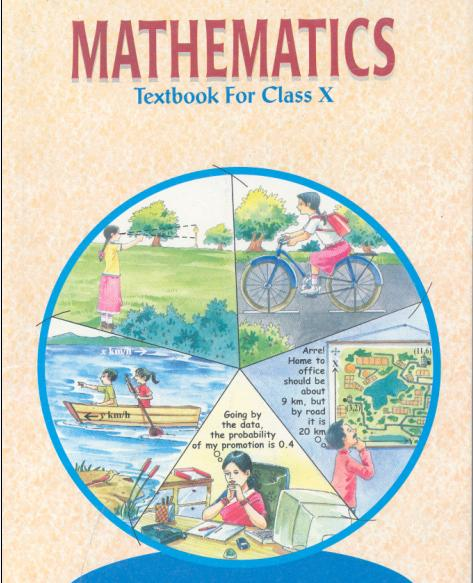## NCERT Solutions for Class 10 Maths Quadratic Equations

###### 1. Check whether the following are Quadratic Equations.

(i)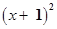= 2 (x − 3)

(ii)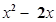= (−2) (3 − x)

(iii) (x − 2) (x + 1) = (x − 1) (x + 3)

(iv) (x − 3) (2x + 1) = x (x + 5)

(v) (2x − 1) (x − 3) = (x + 5) (x − 1)

(vi)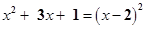(vii)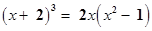(viii)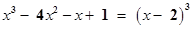Ans. (i)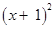= 2 (x − 3)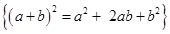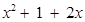= 2x – 6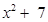= 0

Here, degree of equation is 2.

Therefore, it is a Quadratic Equation.

NCERT Solutions for Class 10 Maths Exercise 4.1

(ii)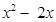= (−2) (3 − x)= −6 + 2x− 2x + 6 = 0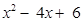= 0

Here, degree of equation is 2.

Therefore, it is a Quadratic Equation.

(iii) (x − 2) (x + 1) = (x − 1) (x + 3)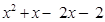=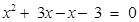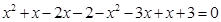x − 2x – 2 − 3x + x + 3 = 0

⇒ −3x + 1 = 0

Here, degree of equation is 1.

Therefore, it is not a Quadratic Equation.

NCERT Solutions for Class 10 Maths Exercise 4.1

(iv) (x − 3) (2x + 1) = x (x + 5)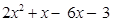=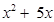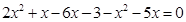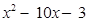= 0

Here, degree of equation is 2.

Therefore, it is a quadratic equation.

(v) (2x − 1) (x − 3) = (x + 5) (x − 1)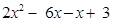=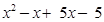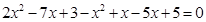x2 − 11x + 8 = 0

Here, degree of Equation is 2.

Therefore, it is a Quadratic Equation.

(vi)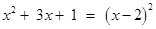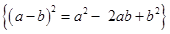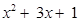=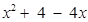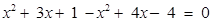⇒ 7x – 3 = 0

Here, degree of equation is 1.

Therefore, it is not a Quadratic Equation.

(vii)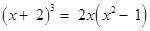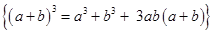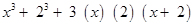=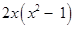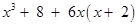=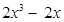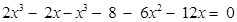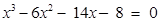Here, degree of Equation is 3.

Therefore, it is not a quadratic Equation.

(viii)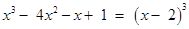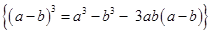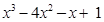=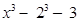(x) (2) (x − 2)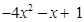=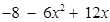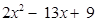= 0

Here, degree of Equation is 2.

Therefore, it is a Quadratic Equation.

NCERT Solutions for Class 10 Maths Exercise 4.1

###### 2. Represent the following situations in the form of Quadratic Equations:

(i) The area of rectangular plot is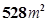. The length of the plot (in metres) is one more than twice its breadth. We need to find the length and breadth of the plot.

(ii) The product of two consecutive numbers is 306. We need to find the integers.

(iii) Rohan’s mother is 26 years older than him. The product of their ages (in years) after 3 years will be 360. We would like to find Rohan’s present age.

(iv) A train travels a distance of 480 km at uniform speed. If, the speed had been 8km/h less, then it would have taken 3 hours more to cover the same distance. We need to find speed of the train.

Ans. (i) We are given that area of a rectangular plot is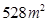.

Let breadth of rectangular plot be x metres

Length is one more than twice its breadth.

Therefore, length of rectangular plot is (2x + 1) metres

Area of rectangle = length × breadth

⇒ 528 = x (2x + 1)

⇒ 528 =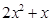– 528 = 0

(ii) Let two consecutive numbers be x and (x + 1).

It is given that x (x + 1) = 306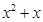= 306– 306 = 0

(iii) Let present age of Rohan = x years

Let present age of Rohan’s mother = (x + 26) years

Age of Rohan after 3 years = (x + 3) years

Age of Rohan’s mother after 3 years = x + 26 + 3 = (x + 29) years

According to given condition:

(x + 3) (x + 29) = 360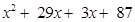= 360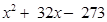= 0

(iv) Let speed of train be x km/h

Time taken by train to cover 480 km = 480x hours

If, speed had been 8km/h less then time taken would be (480x−8) hours

According to given condition, if speed had been 8km/h less then time taken is 3 hours less.

Therefore, 480x – 8 = 480x + 3

⇒ 480 (1x – 8 − 1x) = 3

⇒ 480 (x x + 8) (x) (x − 8) = 3

⇒ 480 × 8 = 3 (x) (x − 8)

⇒ 3840 =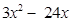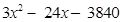= 0

Dividing equation by 3, we get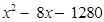= 0

## NCERT Solutions for Class 10 Maths Exercise 4.1

NCERT Solutions Class 10 Maths PDF (Download) Free from myCBSEguide app and myCBSEguide website. Ncert solution class 10 Maths includes text book solutions from Mathematics Book. NCERT Solutions for CBSE Class 10 Maths have total 15 chapters. 10 Maths NCERT Solutions in PDF for free Download on our website. Ncert Maths class 10 solutions PDF and Maths ncert class 10 PDF solutions with latest modifications and as per the latest CBSE syllabus are only available in myCBSEguide.

## CBSE app for Class 10

To download NCERT Solutions for Class 10 Maths, Computer Science, Home Science,Hindi ,English, Social Science do check myCBSEguide app or website. myCBSEguide provides sample papers with solution, test papers for chapter-wise practice, NCERT solutions, NCERT Exemplar solutions, quick revision notes for ready reference, CBSE guess papers and CBSE important question papers. Sample Paper all are made available through the best app for CBSE students and myCBSEguide website.Test Generator

Create question paper PDF and online tests with your own name & logo in minutes.myCBSEguide

Question Bank, Mock Tests, Exam Papers, NCERT Solutions, Sample Papers, Notes

### 6 thoughts on “NCERT Solutions for Class 10 Maths Exercise 4.1”

1. Very useful. Thanks for my CBSE guide team

2. Verry useful

3. The 2(iii) i got xsquare +26x+357=0 which is also is a quadratic eqn so is it correct???????????????????????????????????

4. It’s a good site

5. Thanks?

6. I like this site it’s help me a lot. ??????